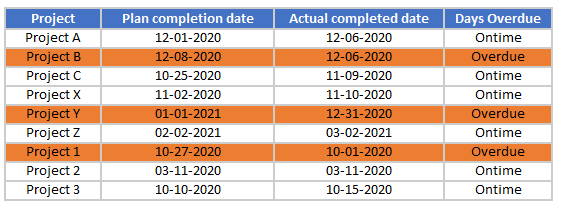# Conditional Formatting Formulas Not Working? – Excel & Google Sheets

This tutorial will demonstrate how to test formulas before applying them to Conditional Formatting in Excel.

## Test Custom Formulas

When applying a custom formula to conditional formatting in Excel, it is important to make sure that the formula actually returns the correct TRUE or FALSE value in your worksheet for the conditional formatting to work correctly.

Prior to creating the conditional formatting rule, you can create the formula in a free space in the workbook and make sure the formula works correctly.

### Test Formula to Highlight Cell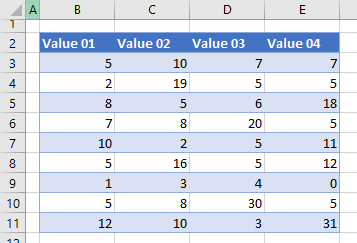In the example above, you wish to write a formula to see if each cell in the worksheet is greater than 5 and if it is greater than 5, you wish to color the background of the cell.

1. Start with the first cell in the range – in this case B3, and you can then test each row and column of the range – down to E11.
Starting with a simple formula, you can see that B3 has a value of 5 and is therefore NOT greater than 5.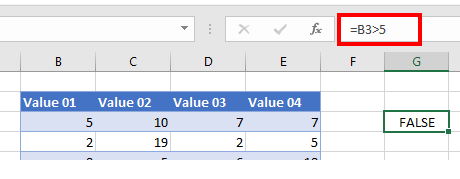1. Then, use the handle to drag that formula down to Row 11, noting that the row part of the cell address (i.e., 3) will change to the next number as you drag the formula down through the rows so B3 becomes B4, then B5 and so on.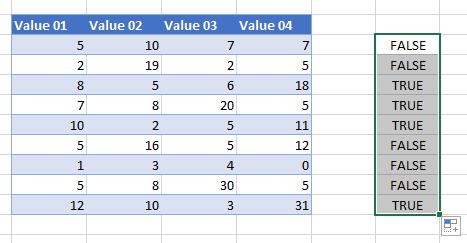1. Then, drag the highlighted range of cells across four columns to test to see if the cells in Columns C to E have a value greater than 5. As you drag the formula across, the column part of the cell address will change accordingly – from Column C to D to E to F.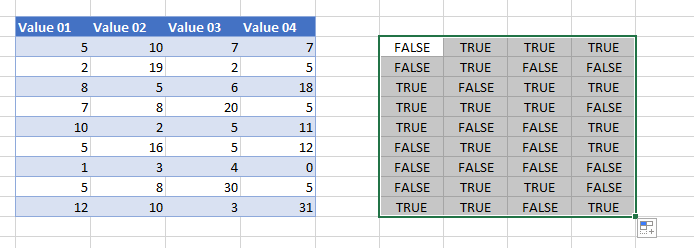1. As you can now see, you get the correct TRUE or FALSE value depending on the value in the corresponding cell.
This confirms the formula is correct and you can use that formula to create the conditional formatting rule. As with the test formula, you use the first cell in the range, B3.
``=B3>5``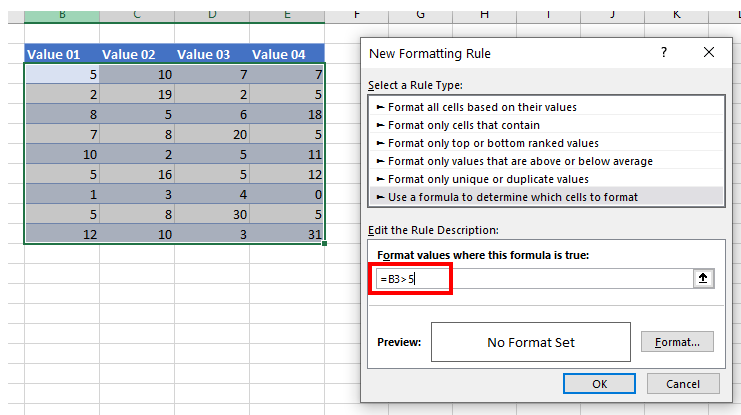1. Once you have typed in the formula, you can set the format for the background color and click OK.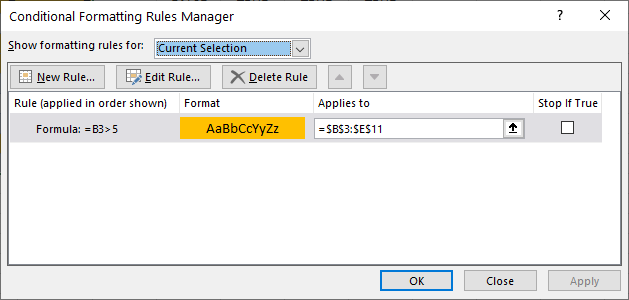As you can see the formula =B3>5 applies to the range B3:E11. The formula cell must always correspond to the first cell in the range to be formatted.

1. Click Apply to apply the formatting to your worksheet.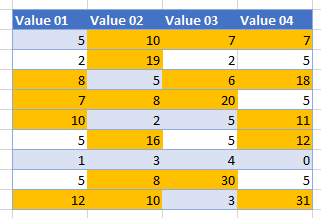### Test Formula to Highlight Row

Applying a formula to change the background color of a row instead of a column is slightly different and a bit more complicated.

Consider the following worksheet.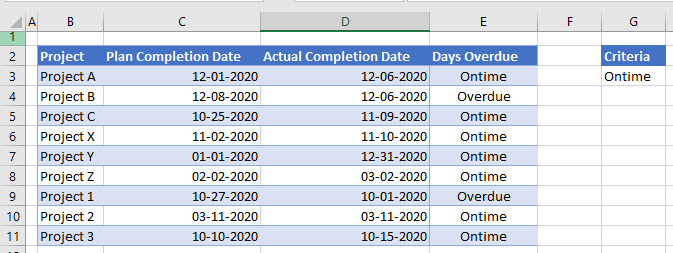In this worksheet, you wish to highlight an entire row if the Project is Overdue – so if Column E has a cell that returns the value Overdue instead of Ontime, you then wish to highlight the entire row that that cell is in.

The formula (using the IF Function) to do that is simple:

``=IF(E4="Overdue", TRUE, FALSE)``

However, if you apply this to the conditional formatting, it returns the following.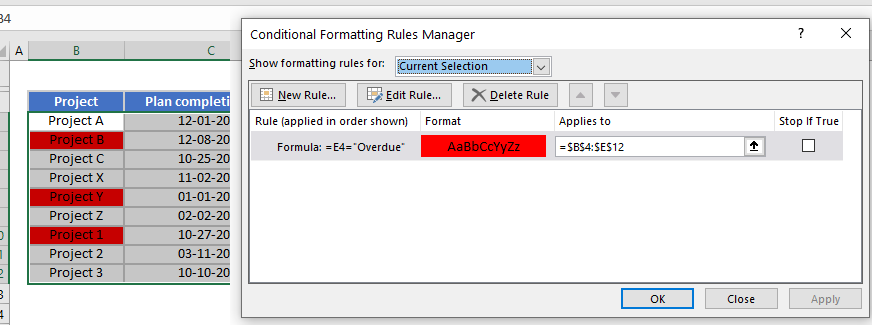Only the first column in the range is formatted.

Now try this formula in your worksheet.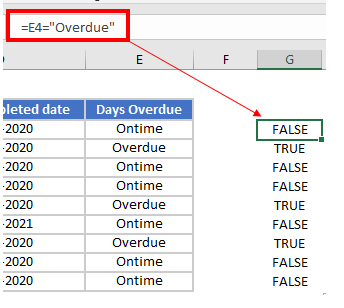You get the desired result: TRUE for when the Project is Overdue in Column E. However, if you were to copy this formula across for the five columns in the range (Column B to Column E), the result returned would be FALSE.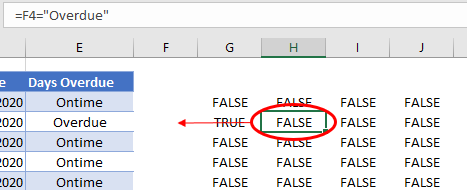The formula would change – so Column E would change to Column F – and of course, there is nothing in Column F, so the formula would return a FALSE every time.

Make sure that the formula ONLY looks at Column E – but that is looks at the correct row – so when you copy the formula across, Column E stays the same. To do that, use a mixed reference that locks the column in place.

``=\$E4="OverDue"``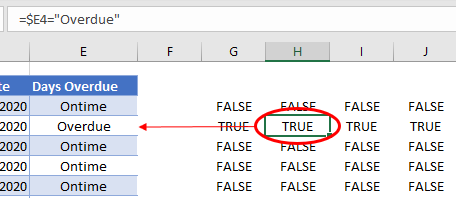Now, when you copy it over four columns, the column in the formula will stay the same, but the row will change.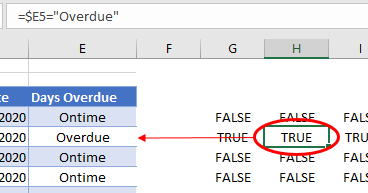With the formula now working in the worksheet, you can create a custom formula in Conditional Formatting.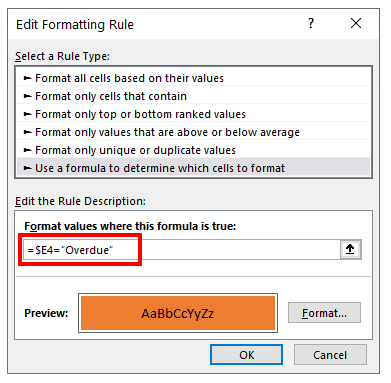When you click OK, then Apply, rows that have Column E Overdue are highlighted.